# Separate Strings

To separate strings in Excel, use RIGHT, LEN, FIND and LEFT. If you're not a formula hero, use Flash Fill to split text strings in Excel.

### Formulas

The problem we are dealing with is that we need to tell Excel where we want to separate the string (see picture below).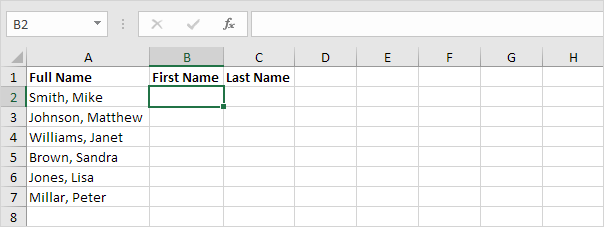In case of Smith, Mike the comma is at position 6 while in case of Williams, Janet the comma is at position 9.

1. To get the first name, use the formula below.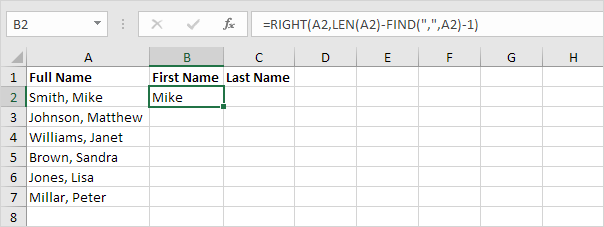Explanation: to find the position of the comma, use the FIND function (position 6). To get the length of a string, use the LEN function (11 characters). =RIGHT(A2,LEN(A2)-FIND(",",A2)-1) reduces to =RIGHT(A2,11-6-1). =RIGHT(A2,4) extracts the 4 rightmost characters and gives the desired result (Mike).

2. To get the last name, use the following formula.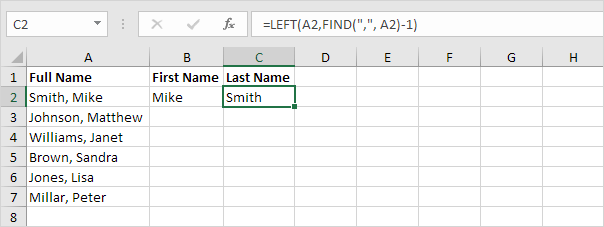Explanation: to find the position of the comma, use the FIND function (position 6). =LEFT(A2,FIND(",", A2)-1) reduces to =LEFT(A2,6-1). =LEFT(A2,5) extracts the 5 leftmost characters and gives the desired result (Smith).

3. Select the range B2:C2 and drag it down.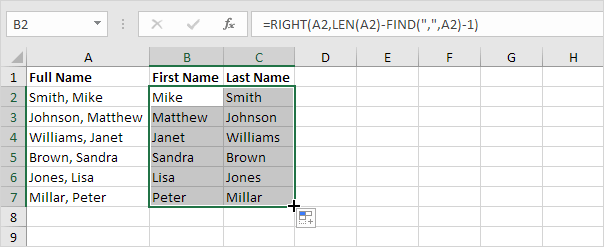There's an even quicker way to achieve this result. Read on.

### Flash Fill

If you're not a formula hero, use Flash Fill to split text strings in Excel.

1. First, tell Excel what you want to do by entering the first name Mike into cell B2.2. On the Data tab, in the Data Tools group, click Flash Fill (or press CTRL + E).Result: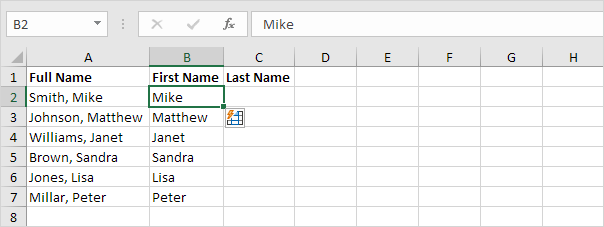That's pretty cool!

3. Next, enter the last name Smith into cell C2.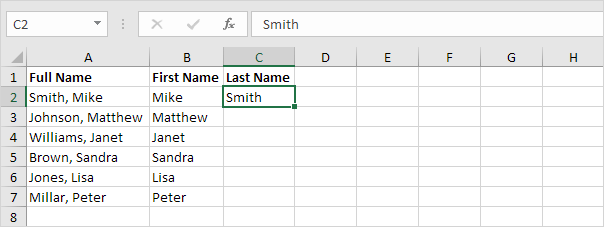4. On the Data tab, in the Data Tools group, click Flash Fill (or press CTRL + E).Result: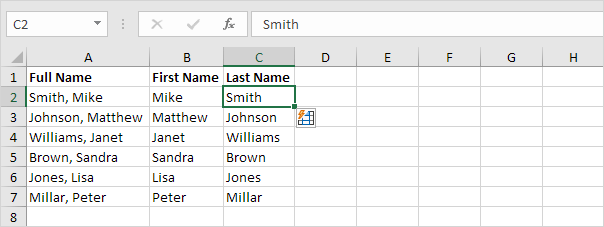Note: one drawback when using Flash Fill is that the output will not automatically update when the source data changes. Create formulas (see first paragraph) to overcome this limitation.

### TextSplit

If you have Excel 365, use the new TEXTSPLIT function to split text into rows or columns using delimiters.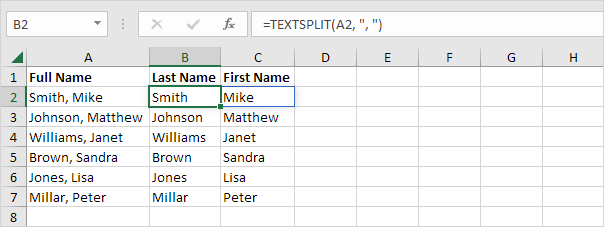Note: the TEXTSPLIT function, entered into cell B2, fills multiple cells (B2 and C2). Wow! This behavior is called spilling.